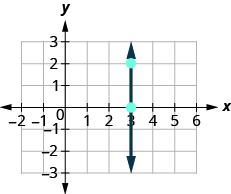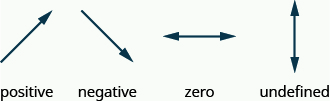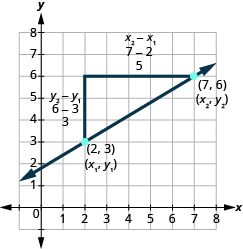# 4.4 Understand slope of a line  (Page 4/14)

 Page 4 / 14

The floor of your room is horizontal. Its slope is 0. If you carefully placed a ball on the floor, it would not roll away.

Now, we’ll consider a vertical line, the line.$\begin{array}{ccccc}\text{What is the rise?}\hfill & & \phantom{\rule{5em}{0ex}}& & \text{The rise is 2.}\hfill \\ \text{What is the run?}\hfill & & \phantom{\rule{5em}{0ex}}& & \text{The run is 0.}\hfill \\ \text{What is the slope?}\hfill & & \phantom{\rule{5em}{0ex}}& & \begin{array}{ccc}\hfill m& =\hfill & \frac{\text{rise}}{\text{run}}\hfill \\ \hfill m& =\hfill & \frac{2}{0}\hfill \end{array}\hfill \end{array}$

But we can’t divide by 0. Division by 0 is not defined. So we say that the slope of the vertical line $x=3$ is undefined.

The slope of any vertical line is undefined. When the x -coordinates of a line are all the same, the run is 0.

## Slope of a vertical line

The slope of a vertical line, $x=a$ , is undefined.

Find the slope of each line:

$x=8$ $y=-5$ .

## Solution

$x=8$
This is a vertical line.
Its slope is undefined.

$y=-5$
This is a horizontal line.
It has slope 0.

Find the slope of the line: $x=-4.$

undefined

Find the slope of the line: $y=7.$

0

## Quick guide to the slopes of linesRemember, we ‘read’ a line from left to right, just like we read written words in English.

## Use the slope formula to find the slope of a line between two points

Doing the Manipulative Mathematics activity “Slope of Lines Between Two Points” will help you develop a better understanding of how to find the slope of a line between two points.

Sometimes we’ll need to find the slope of a line between two points when we don’t have a graph to count out the rise and the run. We could plot the points on grid paper, then count out the rise and the run, but as we’ll see, there is a way to find the slope without graphing. Before we get to it, we need to introduce some algebraic notation.

We have seen that an ordered pair $\left(x,y\right)$ gives the coordinates of a point. But when we work with slopes, we use two points. How can the same symbol $\left(x,y\right)$ be used to represent two different points? Mathematicians use subscripts to distinguish the points.

$\begin{array}{ccc}\left({x}_{1},{y}_{1}\right)\hfill & & \text{read ‘}\phantom{\rule{0.2em}{0ex}}x\phantom{\rule{0.2em}{0ex}}\text{sub 1,}\phantom{\rule{0.2em}{0ex}}y\phantom{\rule{0.2em}{0ex}}\text{sub 1’}\hfill \\ \left({x}_{2},{y}_{2}\right)\hfill & & \text{read ‘}\phantom{\rule{0.2em}{0ex}}x\phantom{\rule{0.2em}{0ex}}\text{sub 2,}\phantom{\rule{0.2em}{0ex}}y\phantom{\rule{0.2em}{0ex}}\text{sub 2’}\hfill \end{array}$

The use of subscripts in math is very much like the use of last name initials in elementary school. Maybe you remember Laura C. and Laura M. in your third grade class?

We will use $\left({x}_{1},{y}_{1}\right)$ to identify the first point and $\left({x}_{2},{y}_{2}\right)$ to identify the second point.

If we had more than two points, we could use $\left({x}_{3},{y}_{3}\right)$ , $\left({x}_{4},{y}_{4}\right)$ , and so on.

Let’s see how the rise and run relate to the coordinates of the two points by taking another look at the slope of the line between the points $\left(2,3\right)$ and $\left(7,6\right)$ .Since we have two points, we will use subscript notation, $\left(\stackrel{{x}_{1},}{2,}\stackrel{{y}_{1}}{3}\right)$ $\left(\stackrel{{x}_{2},{y}_{2}}{7,6}\right)$ .

On the graph, we counted the rise of 3 and the run of 5.

Notice that the rise of 3 can be found by subtracting the y -coordinates 6 and 3.

$3=6-3$

And the run of 5 can be found by subtracting the x -coordinates 7 and 2.

$5=7-2$

We know $m=\frac{\text{rise}}{\text{run}}$ . So $m=\frac{3}{5}$ .

We rewrite the rise and run by putting in the coordinates $m=\frac{6-3}{7-2}$ .

But 6 is ${y}_{2}$ , the y -coordinate of the second point and 3 is ${y}_{1}$ , the y -coordinate of the first point.

So we can rewrite the slope using subscript notation. $m=\frac{{y}_{2}-{y}_{1}}{7-2}$

Also, 7 is ${x}_{2}$ , the x -coordinate of the second point and 2 is ${x}_{1}$ , the x -coordinate of the first point.

So, again, we rewrite the slope using subscript notation. $m=\frac{{y}_{2}-{y}_{1}}{{x}_{2}-{x}_{1}}$

We’ve shown that $m=\frac{{y}_{2}-{y}_{1}}{{x}_{2}-{x}_{1}}$ is really another version of $m=\frac{\text{rise}}{\text{run}}$ . We can use this formula to find the slope of a line when we have two points on the line.

What is the lcm of 340
How many numbers each equal to y must be taken to make 15xy
15x
Martin
15x
Asamoah
15x
Hugo
1y
Tom
1y x 15y
Tom
find the equation whose roots are 1 and 2
(x - 2)(x -1)=0 so equation is x^2-x+2=0
Ranu
I believe it's x^2-3x+2
NerdNamedGerg
because the X's multiply by the -2 and the -1 and than combine like terms
NerdNamedGerg
find the equation whose roots are -1 and 4
Ans = ×^2-3×+2
Gee
find the equation whose roots are -2 and -1
(×+1)(×-4) = x^2-3×-4
Gee
there's a chatting option in the app wow
Nana
That's cool cool
Nana
Nice to meet you all
Nana
you too.
Joan
😃
Nana
Hey you all there are several Free Apps that can really help you to better solve type Equations.
Debra
Debra, which apps specifically. ..?
Nana
am having a course in elementary algebra ,any recommendations ?
samuel
Samuel Addai, me too at ucc elementary algebra as part of my core subjects in science
Nana
me too as part of my core subjects in R M E
Ken
at ABETIFI COLLEGE OF EDUCATION
Ken
ok great. Good to know.
Joan
5x + 1/3= 2x + 1/2
sanam
Plz solve this
sanam
5x - 3x = 1/2 - 1/3 2x = 1/6 x = 1/12
Ranu
Thks ranu
sanam
a trader gains 20 rupees loses 42 rupees and then gains ten rupees Express algebraically the result of his transactions
a trader gains 20 rupees loses 42 rupees and then gains 10 rupees Express algebraically the result of his three transactions
vinaya
a trader gains 20 rupees loses 42 rupees and then gains 10 rupees Express algebraically the result of his three transactions
vinaya
a trader gains 20 rupees loses 42 rupees and then gains 10 rupees Express algebraically the result of his three transactions
vinaya
Kim is making eight gallons of punch from fruit juice and soda. The fruit juice costs $6.04 per gallon and the soda costs$4.28 per gallon. How much fruit juice and how much soda should she use so that the punch costs $5.71 per gallon? Mohamed Reply (a+b)(p+q+r)(b+c)(p+q+r)(c+a) (p+q+r) muhammad Reply 4x-7y=8 2x-7y=1 what is the answer? Ramil Reply x=7/2 & y=6/7 Pbp x=7/2 & y=6/7 use Elimination Debra true bismark factoriz e usman 4x-7y=8 X=7/4y+2 and 2x-7y=1 x=7/2y+1/2 Peggie Ok cool answer peggie Frank thanks Ramil copy and complete the table. x. 5. 8. 12. then 9x-5. to the 2nd power+4. then 2xto the second power +3x Sandra Reply What is c+4=8 Penny Reply 2 Letha 4 Lolita 4 Rich 4 thinking C+4=8 -4 -4 C =4 thinking I need to study Letha 4+4=8 William During two years in college, a student earned$9,500. The second year, she earned $500 more than twice the amount she earned the first year. Nicole Reply 9500=500+2x Debra 9500-500=9000 9000÷2×=4500 X=4500 Debra X + Y = 9500....... & Y = 500 + 2X so.... X + 500 + 2X = 9500, them X = 3000 & Y = 6500 Pbp Bruce drives his car for his job. The equation R=0.575m+42 models the relation between the amount in dollars, R, that he is reimbursed and the number of miles, m, he drives in one day. Find the amount Bruce is reimbursed on a day when he drives 220 miles. Josh Reply Reiko needs to mail her Christmas cards and packages and wants to keep her mailing costs to no more than$500. The number of cards is at least 4 more than twice the number of packages. The cost of mailing a card (with pictures enclosed) is $3 and for a package the cost is$7.
hey
Juan
Sup
patrick
The sum of two numbers is 155. The difference is 23. Find the numbers
The sum of two numbers is 155. Their difference is 23. Find the numbers
Michelle
The difference between 89 and 66 is 23
Ciid
Joy is preparing 20 liters of a 25% saline solution. She only has 40% and 10% solution in her lab. How many liters of the 40% and how many liters of the 10% should she mix to make the 25% solution?
hello
bismark
I need a math tutor BAD
Stacie
Me too
Letha
me too
Xavier
ok
Bishal
teet
Bishal
my answer is y10 that right
mistyByByByByBy